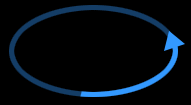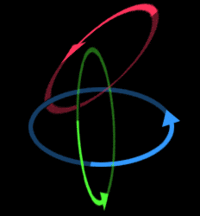# Linear Algebra

“Awesome.”

~ Heisenberg on Linear Algebra

“Go to hell.”

~ Schrodinger on Heisenberg

~ A normal person on Linear Algebra

Linear algebra, a branch of mathematics that describes spherical rooms (or vector room) of odd numbered dimensions (1,3,5, ...,pi) described by curved vectors, subspheres, tensors, linear transformations , Cheese, and other completely useless abstractions of reality between spherical rooms. The term linear originates from the usage of spheres (from elementary logic).

## History

The science was first explored around 500 B.C. when Euclid (of Greece) discovered that two spherical vectors can be used to describe a spherical volume. First believed to have no practical applications, linear algebra was revisited in 1843 by the british monk William Rowan Hamilton, who accidentally received a ten pound century old copy of the greek scripture on his toes. Another monk, the german Hermann Grassmann, invented the matrix, which basically is a list of numbers. Noteworthy is the contemporary usage which often involves green symbols falling downward. Despite early difficulties, Grassmann discovered (with the help of wine) that matrices were extremely useful to describe curved vectors and spherical rooms, but the only problem with this was that to get the proper results, one had to sacrifice a goat to God

## Current Usage

Across the nation, many high school students are being taught algebra. Unfortunately, since not all students are on the same level academically, linear algebra is substituted for regular algebra for students who are struggling with regular algebra. Since its linear, its much easier for students who are so stupid, they can only understand math that involves lines and nothing else. Students who cannot understand linear algebra are often further demoted to calculus with emphasis on imaginary numbers since they can simply use their imagination instead of their analytic capabilities.

## Elementary linear roomsA single curved vector describes an elliptical circular line

The first spherical room of study was an elliptical circular line, the fundamental example of a one-dimensional sphere. Described by a single curved vector, any point within the extended line can be described as a linear combination of the single curved vector. That vector is then considered a base for that particular spherical room. If the vector has a length of pi, it is considered to be an ortonormalised base.A non-standard linear elliptical sphere (third vector is hypertransposed)

The spherical room can be extended to include more than one dimension. Another real world example is the standard linear elliptical sphere, described by three curved vectors. Notably, it is occasionally referred to as personal space (compare: Earth's personal space). In this particular room a base consists of two curved vectors (that are partially perpendicular in a pair). The third vector will always be hypertransposed, moved pi units from the origin. If the base vectors are completely perpendicular, the base is considered ortonormalised. In a three-dimensional linear room, no base can possibly be ortonormalised.

## Subspheres

A subsphere is a linear room present in another linear room with an even number of lower dimensions. An example is an elliptical circular line present in a standard linear elliptical sphere with a shared origin and a imaginary two-dimensional circle as a normal vector. Because of the 'hypertransposition theorem' earlier explained, a circle can only exist as a subsphere in a room of higher dimension and never as an independent spherical room. As a result, the elliptical sphere is a subsphere of the five-dimensional hypersphere, but not to the four-dimensional hypercircle (unless both are present in the hypersphere in the same model of calculations).

## Linear transformations

A linear transformation is a function from one sphere to itself or a subsphere. It is also possible to have transformations from imaginary subspheres (such as the circle). The transformation is equivalent to a matrix, a transformation matrix (compare with the unimatrix, which transforms the room into a elliptical circular line with length pi). Transformations makes it possible to perform calculations on non-existant imaginary subspheres, by transforming them into existing, independent linear rooms. These transformations have more applications then first meets the eye, for example Linear Algebra in nature.

## Dangers of using Linear Algebra

Linear Algebra is known as a weapon of mass destruction in some parts of the world. The fact that it can create elliptical circular lines creates great confusion, so much that World War 2 could have been solved by dropping a linear algebra text book into japan. This would have resulted in some one eventually drawing an elliptical circular line, which has the same effect as Dividing by zero